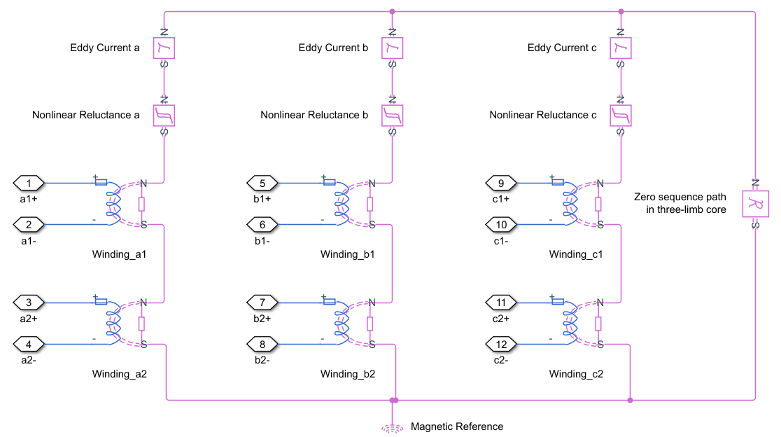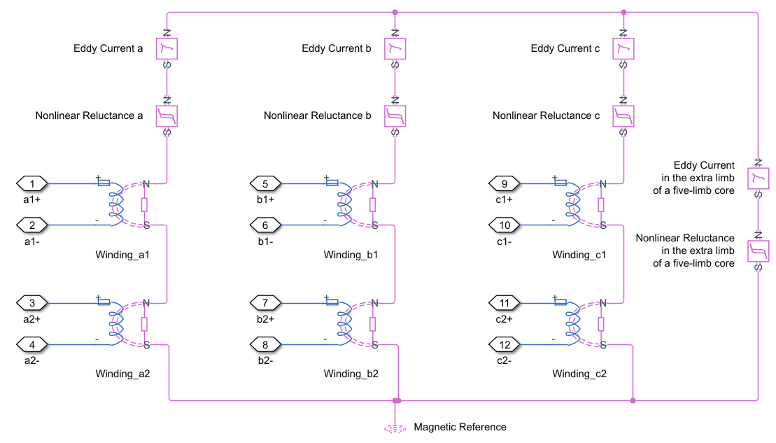# Two-Winding Transformer (Three-Phase)

Three-phase linear nonideal wye- and delta-configurable two-winding transformer with saturation capability

Since R2019a

•Libraries:
Simscape / Electrical / Passive / Transformers

## Description

The Two-Winding Transformer (Three-Phase) block represents a linear nonideal three-phase two-winding transformer that transfers electrical energy between two or more circuits through electromagnetic induction. The block includes linear winding leakage and linear core magnetization effects. You can parameterize the block impedance using per-unit values. The primary and secondary winding types, delta-wye phase angle, and core types are configurable.

The configuration options for both the primary and secondary windings are:

• Wye with floating neutral — Star or T configuration with Floating Neutral (Three-Phase)

• Wye with neutral port — Star or T configuration with Neutral Port (Three-Phase)

• Wye with grounded neutral — Star or T configuration with Grounded Neutral (Three-Phase)

• Delta 1 o'clock — Mesh configuration with a lagging 30 degree phase shift relative to the voltage of a connected wye configuration

• Delta 11 o'clock — Mesh configuration with leading 30 degree phase shift relative to the voltage of a connected wye configuration

Options for the core type are:

• Three-phase five-limb

• Three-phase three-limb

Although a three-limb core is typically less expensive, a five-limb core offers these advantages:

• Lower impedance for the zero-sequence component of current, that is between the line and neutral, in the case of an unbalanced load

• Greater heat dissipation

### Equations

Three-Limb Core

This block is implemented in the magnetic domain using basic magnetic reluctances, windings, and eddy currents blocks.It is important to determine the relation between the electrical domain parameters from the block mask and the magnetic domain parameters used in the model:

• n1 is the number of the primary winding turns.

• n2 is the number of the secondary winding turns.

• Lm is the shunt magnetizing inductance.

• L0 is the zero-sequence inductance.

• Lp is the primary winding leakage inductance.

• Ls is the secondary winding leakage inductance.

• Rm is the shunt magnetizing resistance.

• R is the magnetizing reluctance between phases.

`$R=\frac{{n}_{1}^{2}}{{L}_{m}}$`
• R0 is the zero sequence reluctance.

`${R}_{0}=\frac{1}{3}\frac{{n}_{1}^{2}}{{L}_{0}-{L}_{p}}$`
• Rl1 is the primary winding leakage reluctance.

`$R{l}_{1}=\frac{{n}_{1}^{2}}{{L}_{p}}$`
• Rl2 is the secondary winding leakage reluctance.

`$R{l}_{2}=\frac{{n}_{2}^{2}}{{L}_{s}}$`
• Leddy is the conductance of eddy current loop

`${L}_{eddy}=\frac{{n}_{1}^{2}}{{R}_{m}}$`

For two-winding transformers (three-phase), the coupling between different windings in each phase is identical.

Five-Limb Core

In the case of a five-limb transformer, the extra magnetic flux paths provided by the extra limbs can be represented by zero sequence reluctances, which are originally designed for magnetic paths through the air in the three-limb transformer.In a five-limb model, the magnetic reluctances from the phases to the extra limbs are supposed to be equal to the magnetic reluctances between phases.

`$R={R}_{0}$`

Therefore we deduce:

`$R={R}_{0}=\frac{{n}_{1}^{2}}{{L}_{m}}$`

### Display Options

You can display the transformer per-unit base values in the MATLAB® command window using the block context menu. To display the values, right-click the block and select Electrical > Display Base Values.

### Variables

To set the priority and initial target values for the block variables before simulation, use the Initial Targets section in the block dialog box or Property Inspector. For more information, see Set Priority and Initial Target for Block Variables.

Nominal values provide a way to specify the expected magnitude of a variable in a model. Using system scaling based on nominal values increases the simulation robustness. You can specify nominal values using different sources, including the Nominal Values section in the block dialog box or Property Inspector. For more information, see System Scaling by Nominal Values.

## Ports

### Conserving

expand all

Expandable three-phase electrical conserving port associated with the three-phase, [a1 b1 c1], voltage of winding 1.

Electrical conserving port associated with the primary winding neutral point.

#### Dependencies

This port is only visible when the Main parameter Winding 1 connection type is set to `Wye with neutral port`.

Expandable three-phase electrical conserving port associated with the three-phase, [a2 b2 c2], voltage of the first secondary winding.

Electrical conserving port associated with the first secondary winding neutral point.

#### Dependencies

This port is only visible when the Main parameter Winding 2 connection type is set to `Wye with neutral port`.

## Parameters

expand all

Whether to model composite or expanded three-phase ports.

Composite three-phase ports represent three individual electrical conserving ports with a single block port. You can use composite three-phase ports to build models that correspond to single-line diagrams of three-phase electrical systems.

Expanded three-phase ports represent the individual phases of a three-phase system using three separate electrical conserving ports.

### Main

Apparent power flowing through the transformer when operating at rated capacity. The value must be greater than `0`.

Rated or nominal frequency of the AC network to which the transformer is connected. The value must be greater than `0`.

Primary winding type.

RMS line voltage applied to the primary winding under normal operating conditions. The value must be greater than `0`.

Secondary winding type.

RMS line voltage applied to the secondary winding under normal operating conditions. The value must be greater than `0`.

Number of limbs that comprise the magnetic circuit.

### Impedances

Per-unit power loss in the primary winding. The value must be greater than `0`.

Per-unit power loss in the secondary winding. The value must be greater than `0`.

Whether to model magnetic flux losses.

Per-unit magnetic flux loss in the primary winding. The value must be greater than `0`.

#### Dependencies

To enable this parameter, set Leakage reactance to `Include`.

Per-unit magnetic flux loss in the secondary winding. The value must be greater than `0`.

#### Dependencies

To enable this parameter, set Leakage reactance to `Include`.

Whether to model the transformer core losses.

Per-unit losses in the transformer core. The value must be greater than `0`.

#### Dependencies

To enable this parameter, set Magnetizing resistance to `Include`.

Whether to model magnetic effects of the transformer core.

Choose if and how you want to represent the magnetic saturation.

#### Dependencies

To enable this parameter, set Magnetizing reactance to `Include`.

Per unit vector of currents. The first value must be `0`. This parameter must be strictly ascending.

#### Dependencies

To enable this parameter, set Magnetizing reactance to `Include` and Magnetic saturation representation to `Lookup table (phi versus i)`.

Per unit vector of magnetic flux. The first value must be `0`. This parameter must be strictly ascending.

#### Dependencies

To enable this parameter, set Magnetizing reactance to `Include` and Magnetic saturation representation to `Lookup table (phi versus i)`.

Per-unit magnetic effects of the transformer core when operating in its linear region. The value must be greater than `0`.

#### Dependencies

To enable this parameter, set Magnetizing reactance to `Include` and Magnetic saturation representation to `None`.

Per-unit zero sequence reactance. The value must be greater than or equal to the primary winding magnetic flux loss.

#### Dependencies

To enable this parameter, set Core type, a Main parameter, to ```Three-phase three-limb```.

## Version History

Introduced in R2019a